CAT  >  Banker's Discount: Important Formulas

# Banker's Discount: Important Formulas - Logical Reasoning (LR) and Data Interpretation (DI) - CAT

 Table of contentsIntroductionBanker's Discount Important formulasImportant ExamplesTips for Banker's Discount##• Suppose merchant A purchases goods worth of saying Rs.5000 from another merchant B at a credit of a certain period say 4 months. Then B draws up a draft i.e.., prepares a special type of bill called "Hundi" or Bill of exchange.
• On the receipt of the goods, A gives an agreement dually signed on the bill stating that he has accepted the bill and money can be withdrawn from his bank account after 4 months of the date of the bill.
• On this bill, there is an order from A to his bank asking to pay Rs.5000 to B after 4 months. Moreover, 3 more days (known as grace days) are added to the date (called nominally due date) of expiry of 4 months, and on the date so obtained (called the legally due date), the bill can be presented to the bank by B to collect Rs.5000 from A‘s account.
• Suppose the bill is drawn on 5th January at 4 months, then the nominally due date is 5th May and the legally due date is 8th May. The amount given on the draft or bill is called the face value which is Rs.5000 in this case.
• Let's say, B needs the money for this bill earlier than 8th May, say, on 3rd March. In such a case, B can sell the bill to a banker or a broker who pays him the money against the bill but somewhat less than the face value.
• Now the natural questions are, as how much cash the banker should pay to B on 3rd March to 8th May, he gains nothing. So in order to make some profit, the banker deducts from the face value. The simple interest on the face value for the unexpired time i.e…, from 3rd March to 8th May. This deduction is known as Banker’s Discount (B.D.) or commercial discount.
• Thus B.D. is the S.I. on face value for the period from the date on which the bill was discounted and the legally due date. The money paid by the banker to the bill holder is called the discountable value.
• Also, the difference between the banker’s discount and the true discount for the unexpired time is called the Banker’s Gain (B.G.). Thus, Banker’s Gain
B.G. = (B.D.) - (T.D.)

Remark: When the date of the bill is not given, grace days are not to be added.

## Banker's Discount Important formulas

• B.D. = S.I. on the bill for unexpired time.
• Banker’s Gain = (B.D.) - (T.D.)
• B.G. = S.I. on T.D.
• T.D.=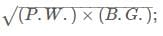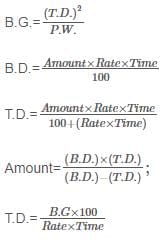## Important Examples

Question 1. The true discount on a bill Rs.1860 due after 8 months is Rs.60. Find the rate, the banker’s discount and the banker’s gain.

Amount = Rs.1860, T.D. = Rs.60
∴ P.W. = Rs.(1860-60) = Rs. 1800
S.I. on Rs. 1800 for 8 months  = Rs.60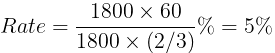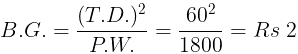B.D. = (T.D.) + (B.G.) = Rs. (60+2) = Rs. 62

Question for Banker's Discount: Important Formulas
Try yourself:The banker's gain of a certain sum due 2 years hence at 10% per annum is Rs. 24. The present worth is:

Question 2. The present worth of a bill due sometime hence is Rs. 1100 and the true discount on the bill is Rs. 110. Find the banker’s discount and the extra gain the banker would make in the transaction.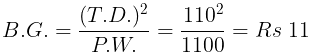∴ B.D. = B.G. + T.D. = Rs. (11+110) = Rs. 121

Question for Banker's Discount: Important Formulas
Try yourself:The banker's gain on a sum due 3 years hence at 12% per annum is Rs. 270. The banker's discount is:

Question 3. The banker’s discount and the true discount on a sum of money due 8 months hence are Rs. 52 and Rs. 50, respectively. Find the sum and the rate per cent.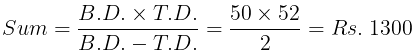∴ B.D. is S.I. on sum due.
⇒ S.I. on Rs. 1300 for 8 months is Rs. 52
Thus,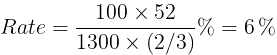Question 4. The banker’s discount on Rs. 1800 at 5% is equal to the true discount on Rs. 1830 for the same time and at the same rate, Find the time.

S.I. on Rs. 1800 = T.D.on Rs. 1830
∴ P.W. of Rs. 1830 is Rs. 1800
i.e., Rs. 30 is S.I. on Rs. 1800 at 5%
∴ Time = (100 × 30)/(1800 × 5) = 1/3 years = 4 months

Question for Banker's Discount: Important Formulas
Try yourself:The present worth of a sum due sometime hence is Rs. 576 and the banker's gain is Rs. 16. The true discount is:

Question 5. If the true discount on a certain sum due 6 months hence at 6% is Rs. 36, what is the banker’s discount on the same sum for the same time and at the same rate ?

B.G.= S.I. on T.D.= Rs.(3600×6×0.5)/100 = Rs.1.08
∴ (B.D.) – (T.D.) = Rs. 1.08
i.e. B.D.=(T.D.)+Rs.1.08 = Rs.(36+1.08) = Rs.37.08

## Tips for Banker's Discount

### Tip #1: Understand the concepts in Banker’s Discount clearly

Scenario: Suppose A has borrowed Rs 1000 from B and this amount should be returned with interest after 1 year. Let us assume that the market interest rate is 5% per year [Simple Interest]. A hands over to B a note with a Face Value of Rs 1050, promising repayment after 1 year. [1000 + (1000 x 0.05 x 1yr) = 1050]

After 6 months, B decides that he needs the money immediately and cannot wait till the due date which is 6 months away. B approaches a bank and hands over the note from A with Face Value of Rs 1050 due after 6 months.

Calculating True Discount:
The present value (or true value) of the note from A is calculated as follows:
PV x (1 + r x t) = FV [Here, PV is the present value, r is the rate of simple interest, t is time and FV is the Face Value of the note.]
Present Value (or True Value) = 1050/(1.025) = Rs. 1024.4
True discount = Face Value – Present Value = 1050 – 1024.4 = Rs. 25.6
But, if the bank paid out Rs 1024.4 to B in exchange for the note, the bank would not make a profit. The bank does not use True Discount but uses another formula to calculate the discount called Banker’s Discount.

Calculating Banker’s Discount:

Banker’s Discount: The Simple Interest on the Face Value of the debt for the time period between the legally due date and the date on which the bill is discounted is called Banker’s Discount.
Banker’s Discount = FV x r x t = 1050 x 0.05 x (1/2) = Rs 26.25
Note:
True Discount = FV – [FV / (1 + r x t)] = FV [r x t / (1 + r x t)] < FV x r x t
True Discount < Banker’s Discount
Instead of discounting True Discount, the Bank discounts the Banker’s Discount from the Face Value and pays out Rs 1050 – 26.25 = Rs. 1023.75
Banker’s Gain = Present Value of the Note – Actual Payout
= (Face Value – True Discount) – (Face Value – Banker’s Discount)
= Banker’s Discount – True Discount [This figure is always positive]

Example 1: The banker's gain on a sum due 3 years hence at 12% per annum is Rs. 270. What is the banker’s discount?
Sol:
Banker’s Discount = FV x r x t = 0.36 x FV
True Discount           = FV – PV = FV – FV / [1 + (r x t)] = FV – FV / 1.36
= 0.36 x FV / 1.36 = Banker’s Discount / 1.36
Banker’s Gain = Banker’s Discount – True Discount = BD - BD/1.36 = 270
⇒ Banker’s Discount, BD = 270 x 1.36 / 0.36 = Rs. 1020

Moving on, we come to the formulae in Banker’s Discounts.

Example 2: The banker's discount on a certain sum due 2 years hence is 11/10 of the true discount. What is the rate?
Sol:
BD = FV x r x t
TD = FV – PV = FV – FV / [1 + (r x t)] = FV x r x t / [1 + (r x t)]
BD/ TD = 1 + (r x t) = 11/10
2r = 1/10
⇒ r = 1/20 = 0.05 or 5%

### Tip #2: For transactions that do not involve a bank, use True Value

Example 1: A man purchased a cow for Rs. 3000 and sold it the same day for Rs. 3600, allowing the buyer a credit of 2 years. If the rate of interest be 10% per annum, then what is his gain?
Sol:
Present Value = 3600/[1+(0.10 x 2)] = Rs 3000
Gain = Present Value – Cost = 0

Example 2A trader owes a merchant Rs. 10,028 due 1 year hence. The trader wants to settle the account after 3 months. If the rate of interest 12% per annum, how much cash should he pay?
Sol:
Face Value = Rs. 10028, r = 12% p.a.
Present Value after 9 months = 10028/[1+(0.12*9/12)] = Rs 9200

The document Banker's Discount: Important Formulas | Logical Reasoning (LR) and Data Interpretation (DI) - CAT is a part of the CAT Course Logical Reasoning (LR) and Data Interpretation (DI).
All you need of CAT at this link: CAT

## Logical Reasoning (LR) and Data Interpretation (DI)

125 videos|165 docs|117 tests

## FAQs on Banker's Discount: Important Formulas - Logical Reasoning (LR) and Data Interpretation (DI) - CAT

 1. What is Banker's Discount?Ans. Banker's Discount is a financial term used in banking and finance to calculate the true discount on a bill of exchange or a promissory note. It is the amount deducted from the face value of the bill when it is paid before maturity.
 2. How is Banker's Discount calculated?Ans. Banker's Discount can be calculated using the formula: Banker's Discount = (Face Value × Rate × Time) / (100 + (Rate × Time)) Where the Face Value is the value of the bill, Rate is the discount rate, and Time is the time period for which the bill is discounted.
 3. What is the difference between Banker's Discount and Simple Interest?Ans. Banker's Discount and Simple Interest are both methods used to calculate the interest on a loan or an investment. However, there are some key differences between the two: - Banker's Discount is used for calculating the discount on bills of exchange or promissory notes, while Simple Interest is used for calculating the interest on loans or investments. - Banker's Discount is based on the face value of the bill, while Simple Interest is based on the principal amount. - Banker's Discount is calculated using the rate and time, while Simple Interest is calculated using the principal, rate, and time.
 4. How is Banker's Discount related to Promissory Notes?Ans. Banker's Discount is closely related to promissory notes. A promissory note is a written promise to pay a certain sum of money to a specified person or bearer at a future date. When a promissory note is discounted by a bank or a financial institution before its maturity, the amount of discount is known as Banker's Discount.
 5. Can Banker's Discount be negative?Ans. No, Banker's Discount cannot be negative. Banker's Discount is always a positive value as it represents the amount deducted from the face value of a bill or a note. If the discount is not applicable or there is no discount, the Banker's Discount will be zero.

## Logical Reasoning (LR) and Data Interpretation (DI)

125 videos|165 docs|117 testsExplore Courses for CAT exam### How to Prepare for CAT

Read our guide to prepare for CAT which is created by Toppers & the best Teachers
Signup to see your scores go up within 7 days! Learn & Practice with 1000+ FREE Notes, Videos & Tests.
10M+ students study on EduRev
Track your progress, build streaks, highlight & save important lessons and more!
Related Searches

,

,

,

,

,

,

,

,

,

,

,

,

,

,

,

,

,

,

,

,

,

;